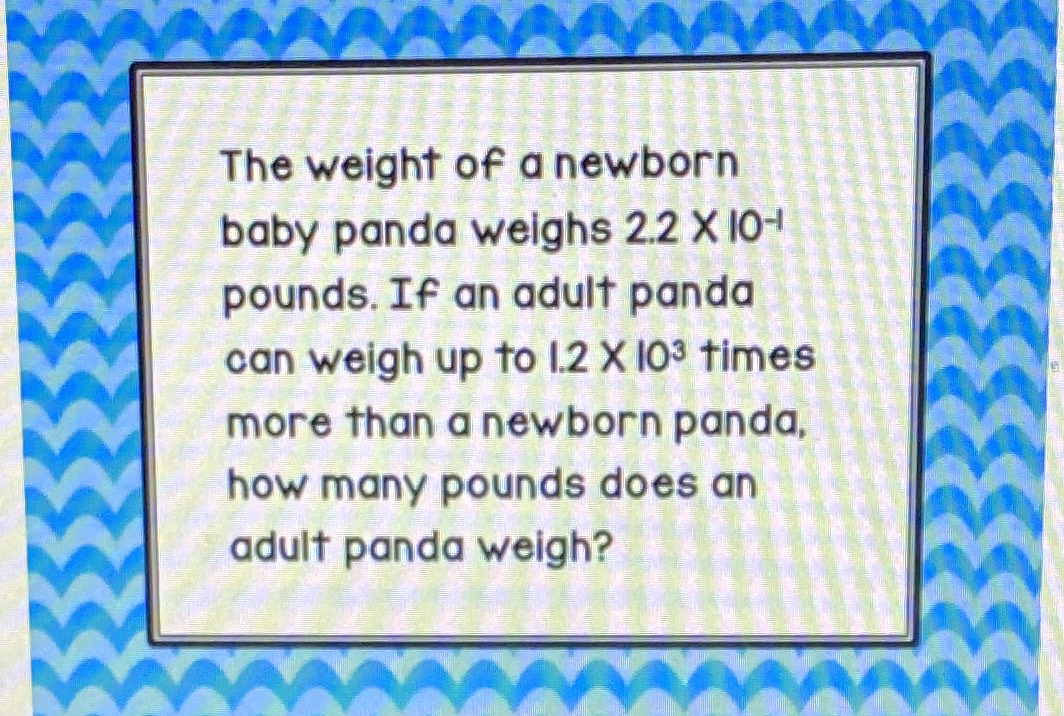¿Todavía tienes preguntas de matemáticas?

Pregunte a nuestros tutores expertos
Arithmetic
QuestionThe weight of a newborn baby panda weighs $$2.2\times 10^{-1}$$ pounds. If an adult panda can weight up to $$1.2\times 10^{3}$$) times more than a newborn panda,how many pounds does an adult panda weight?

$$264$$ pounds